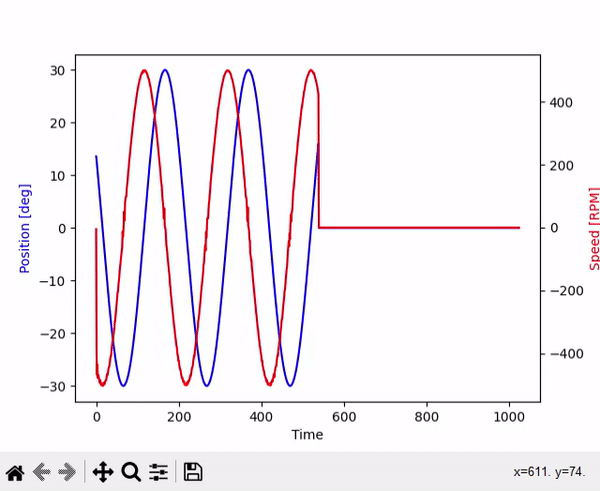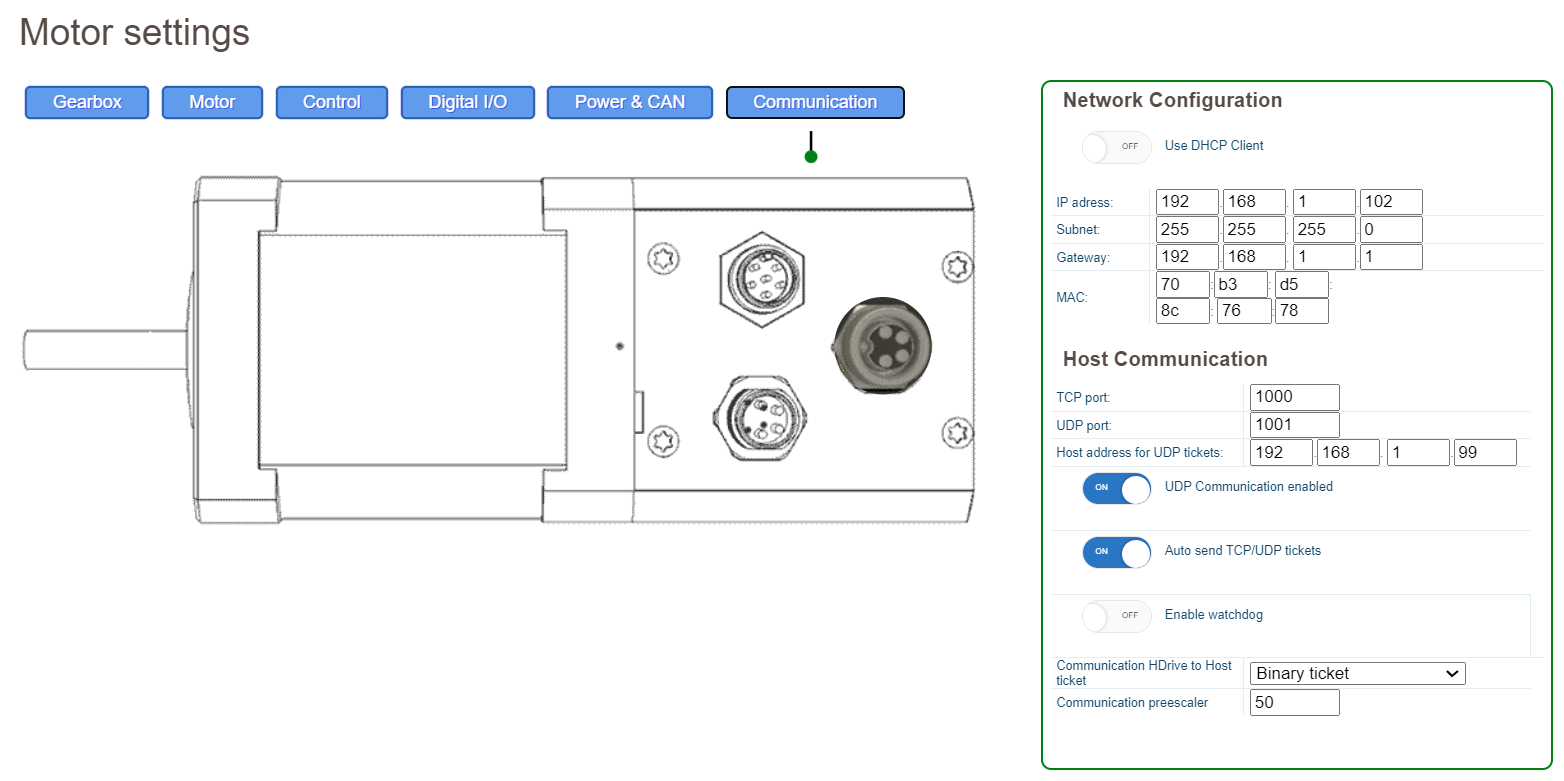## Python Example

The following example shows how to interface the HDrive with Python. The Example plots the current position and speed inside a graph while the motor is running in oscillation mode.## Drive configuration## Enviroment configuration

To draw graphs out of Python, we need to use a plotting library like matplotlib. You can install matplotlib in Python using the following line:

Matplotlib is a plotting library for Python that provides a variety of tools for creating different types of plots, charts, and graphs. It can be used to visualize data in a variety of formats and is widely used in scientific research, data analysis, and visualization. It is a third-party library that can be installed using pip or conda package managers. Install matplotlib with the following line:

`pip install matplotlib`

## Python Code

```import socket
import struct
import time
import signal
import matplotlib.pyplot as plt

# Configure Socket
with socket.socket(socket.AF_INET, socket.SOCK_STREAM) as s:
# Connect to motor
s.connect(('192.168.1.102', 1000))

# Compose String to send to the drive
st = '"<control pos=\"15000\" frequency=\"20\" torque=\"200\" mode=\"135\" offset=\"0\" phase=\"0\" />"'
s.sendall(st.encode('ascii')) # send XML command

while not stop_event.is_set():
time.sleep(1)

index = 0
# Configure Socket
with socket.socket(socket.AF_INET, socket.SOCK_DGRAM) as s:
s.bind(('', udp_port))

while not stop_event.is_set():
data, addr = s.recvfrom(132) # buffer size is 132 bytes
int_list = list(struct.unpack("<33i", data)) # interprete 33 int32 numbers

# Update the ring buffer
ring_buffer[index] = [int_list*1e-3, int_list/10.0, int_list]
index = (index + 1) % len(ring_buffer)

def keyboard_interrupt_handler(signal, frame):
print("Keyboard interrupt received. Stopping the program.")
# Set the stop event to signal the thread to exit
stop_event.set()

# Exit the program
exit(0)

if __name__ == '__main__':
stop_event = threading.Event()  # create an event object

# Initialize the ring buffer
ring_buffer = [[0, 0, 0] for i in range(10000)]

# create a thread that can send commands to the motor

# Install the keyboard interrupt handler
signal.signal(signal.SIGINT, keyboard_interrupt_handler)

# Initialize the plot
plt.ion()
fig, ax = plt.subplots()

while True:

# Get the latest data from the ring buffer
time_values = [entry for entry in ring_buffer]
y_values = [entry for entry in ring_buffer]

# Update the plot with the latest data
ax.clear() # Clear the previous plot
ax.plot(time_values, y_values) # y_values is the list of values you want to plot

ax.set_xlabel('Time') # Set the x-axis label

# Show the plot
plt.pause(0.0001) # Give the plot time to refresh
```